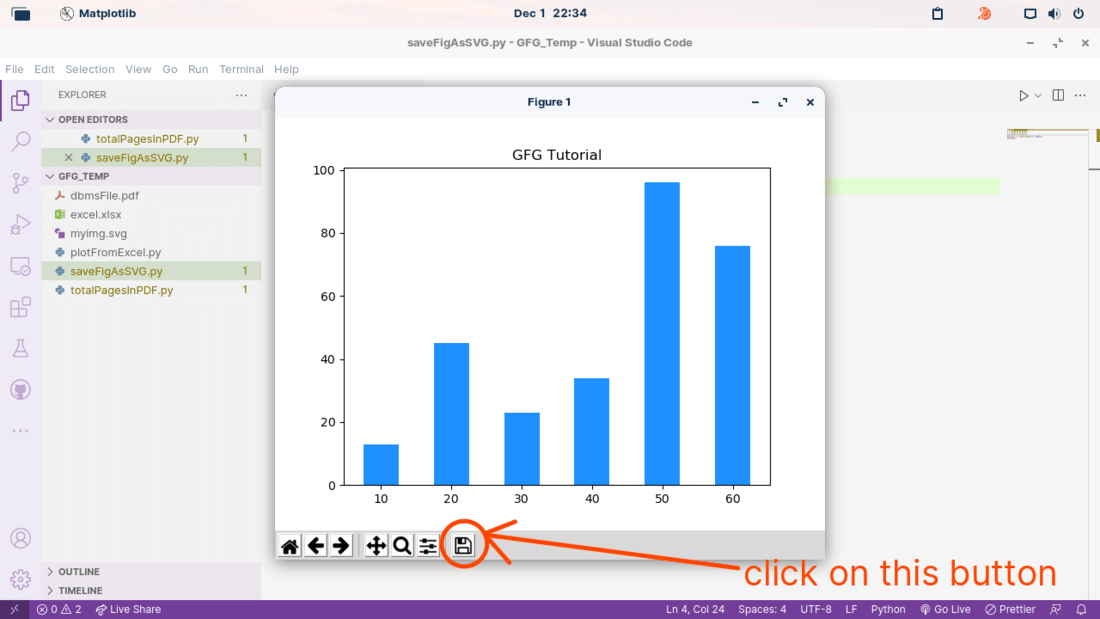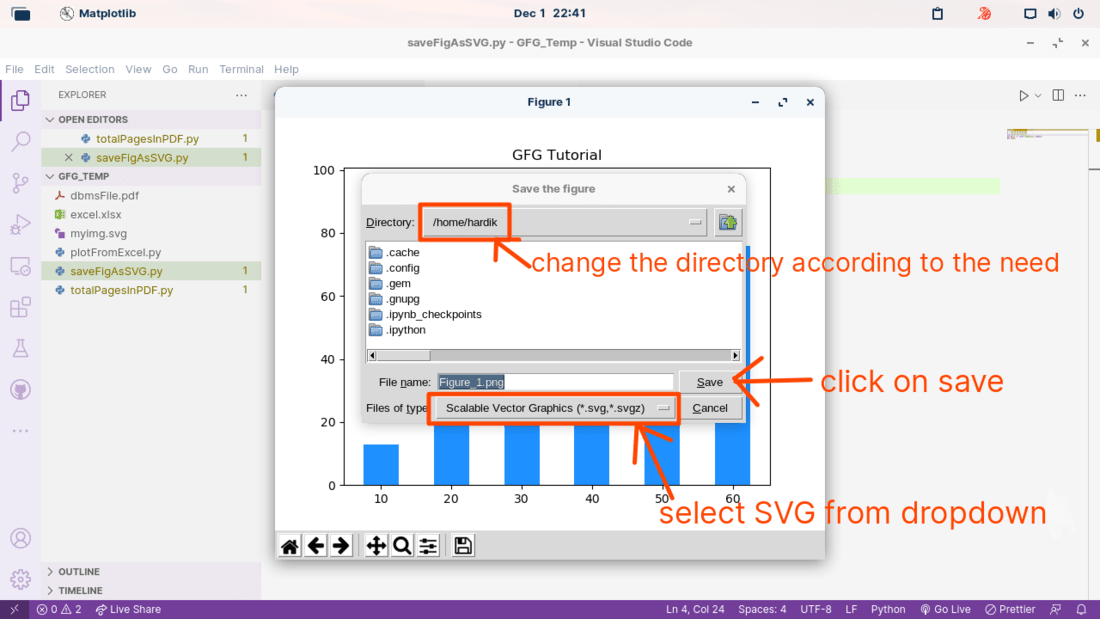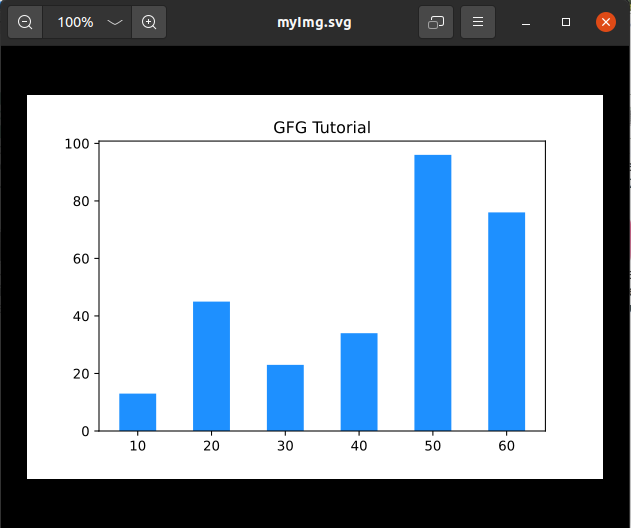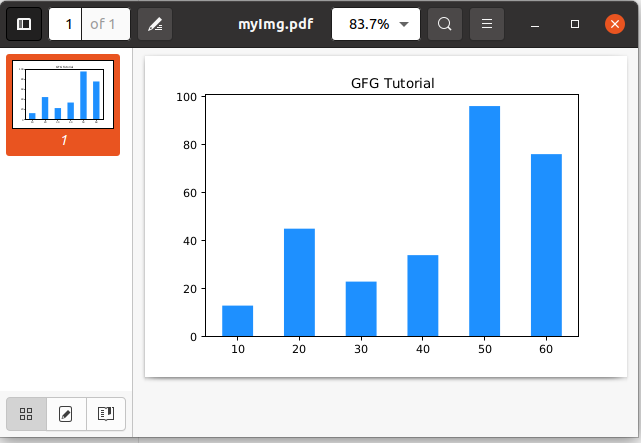Open in App
Not now

# Save Matplotlib Figure as SVG and PDF using Python

• Last Updated : 28 Dec, 2022

In this article, we will see how can we save the Matplotlib figure as Scalable Vector Graphics(SVG) using Python or any other file format for further use. The required modules for this tutorial are Matplotlib. Matplotlib is a comprehensive library for creating static, animated, and interactive visualizations in Python. If you had not installed the Matplotlib library you can install it using the pip command. Here, we will cover two ways to save a Matploblib figure as an SVG:

• Save Matplotlib Figure using GUI Manually
• Save Matplotlib figure using savefig()
• Save the Figure as a PDF

## What is SVG in Python?

SVG stands for Scalable Vector Graphics, It is an XML-based vector image format for defining two-dimensional graphics, having support for interactivity and animation. The SVG specification is an open standard developed by the World Wide Web Consortium in 1999.

### Save Matplotlib Figure using GUI Manually

Here, we imported the matplotlib.pyplot module as plt and then plotted a bar graph by using plt.bar() function for values of the x-axis we used the values of list x and for the y-axis we used the values of list y and set some optional attributes like color and width of bars by using the function arguments color and width, set the title of the graph by using plt.title() function and finally show the figure by using plt.show() function.

## Python3

 `import` `matplotlib.pyplot as plt``x ``=` `[``10``, ``20``, ``30``, ``40``, ``50``, ``60``]``y ``=` `[``13``, ``45``, ``23``, ``34``, ``96``, ``76``]``plt.title(``'GFG Tutorial'``)``plt.bar(x, y, color``=``'dodgerblue'``, width``=``5``)``plt.show()`

Output:

Click on the Save this figure button.Select SVG from the dropdown list, change the directory according to your preference, and hit the Save button.### Save Matplotlib figure using savefig()

First, We imported the matplotlib.pyplot module as plt and then plotted a bar graph by using plt.bar() function for values of the x-axis we used the values of list x and for the y-axis we used the values of list y and set some optional attributes like color and width of bars by using the function arguments color and width, set the title of the graph by using plt.title() function and finally used savefig() method to save the figure as SVG on the desired location.

`plt.savefig('/home/hardik/savedSVG.svg')`

## Python3

 `import` `matplotlib.pyplot as plt``x ``=` `[``10``, ``20``, ``30``, ``40``, ``50``, ``60``]``y ``=` `[``13``, ``45``, ``23``, ``34``, ``96``, ``76``]``plt.title(``'GFG Tutorial'``)``plt.bar(x, y, color``=``'dodgerblue'``, width``=``5``)``plt.savefig(``'/home/hardik/myImg.svg'``)`

Output:### Save the Figure as a PDF

We imported the matplotlib.pyplot module as plt and then plotted a bar graph by using plt.bar() function for values of the x-axis we used the values of list x and for the y-axis we used the values of list y and set some optional attributes like color and width of bars by using the function arguments color and width, set the title of the graph by using plt.title() function and finally used savefig() function to save the figure as pdf on the desired location. To save the figure as pdf we can use the savefig() method in which we can change the file format as pdf like below:

`plt.savefig('filename.pdf')`

## Python3

 `import` `matplotlib.pyplot as plt``x ``=` `[``10``, ``20``, ``30``, ``40``, ``50``, ``60``]``y ``=` `[``13``, ``45``, ``23``, ``34``, ``96``, ``76``]``plt.title(``'GFG Tutorial'``)``plt.bar(x, y, color``=``'dodgerblue'``, width``=``5``)``plt.savefig(``'/home/hardik/myImg.pdf'``)`

Output:My Personal Notes arrow_drop_up